Start typing, then use the up and down arrows to select an option from the list.Jules Bruno
120views
6
now part B of this question, it says, What is the Ph of the solution? This part doesn't require any math on our part. We're dealing with the equivalence point between a strong acid and a strong base, and we've already heard this several times already. At the equivalence point between a strong acid and a strong base, Ph equals exactly seven under normal conditions. I remember when I keep saying normal conditions, all I mean is the temperature is 25 degrees Celsius. Because remember, all these things are connected to K W. 1.0 times 10 to the negative 14. And in our earlier videos we saw that if we change the temperature, we change our K W. And the new definition for neutral would change. It would no longer be seven. So remember the equivalence point for a strong acid and strong base is equal to exactly seven under normal conditions. If it were a weak acid with a strong base or a week faced with a strong acid, the equivalents point ph would be greater than or less than some seven in those cases will learn later on how exactly to find the exact Ph under those different circumstances and this one, we didn't need to do any math because when they're both strong, the answer is exactly seven. Now that we've seen this example, I want you guys to attempt to do the practice, one that's left on the bottom.1:259:00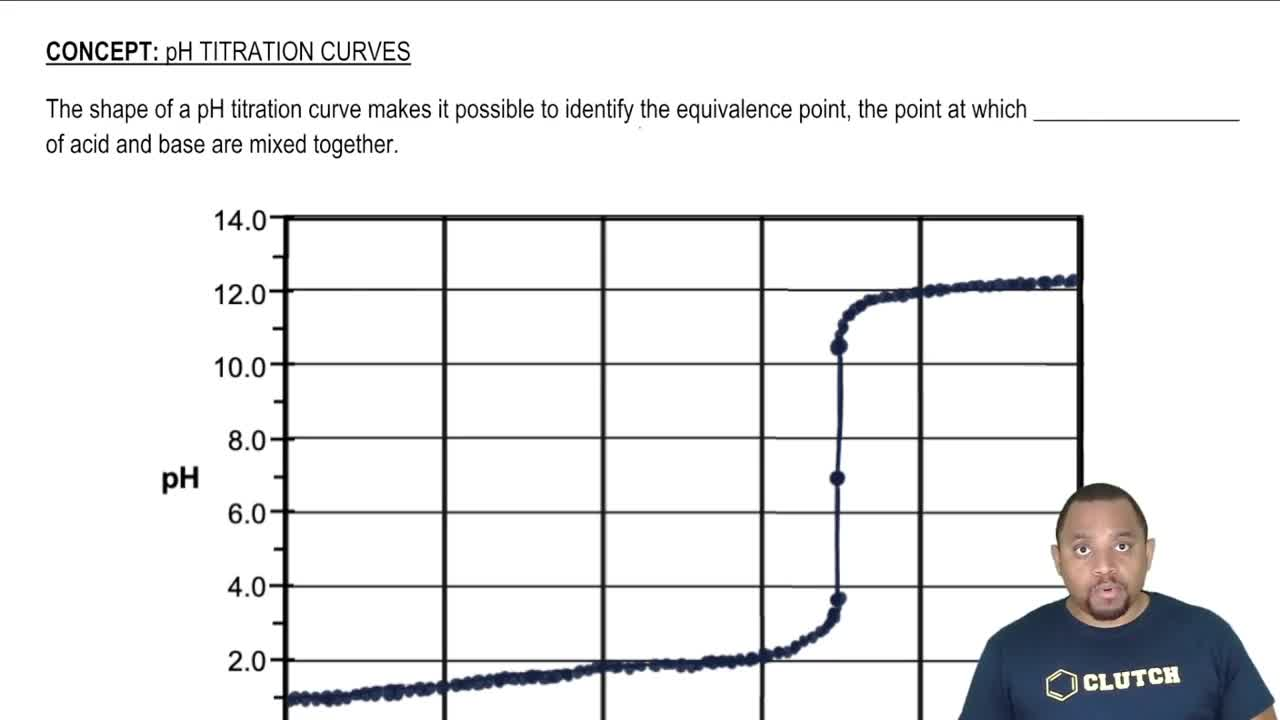04:13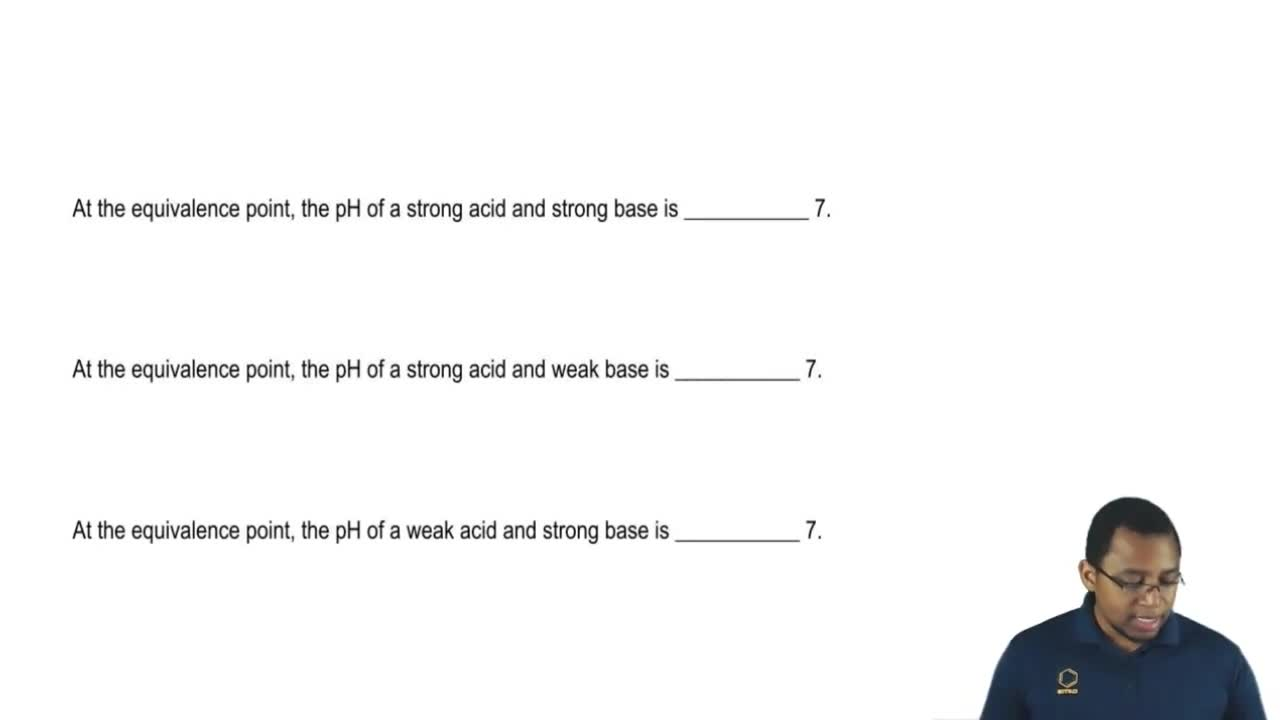01:39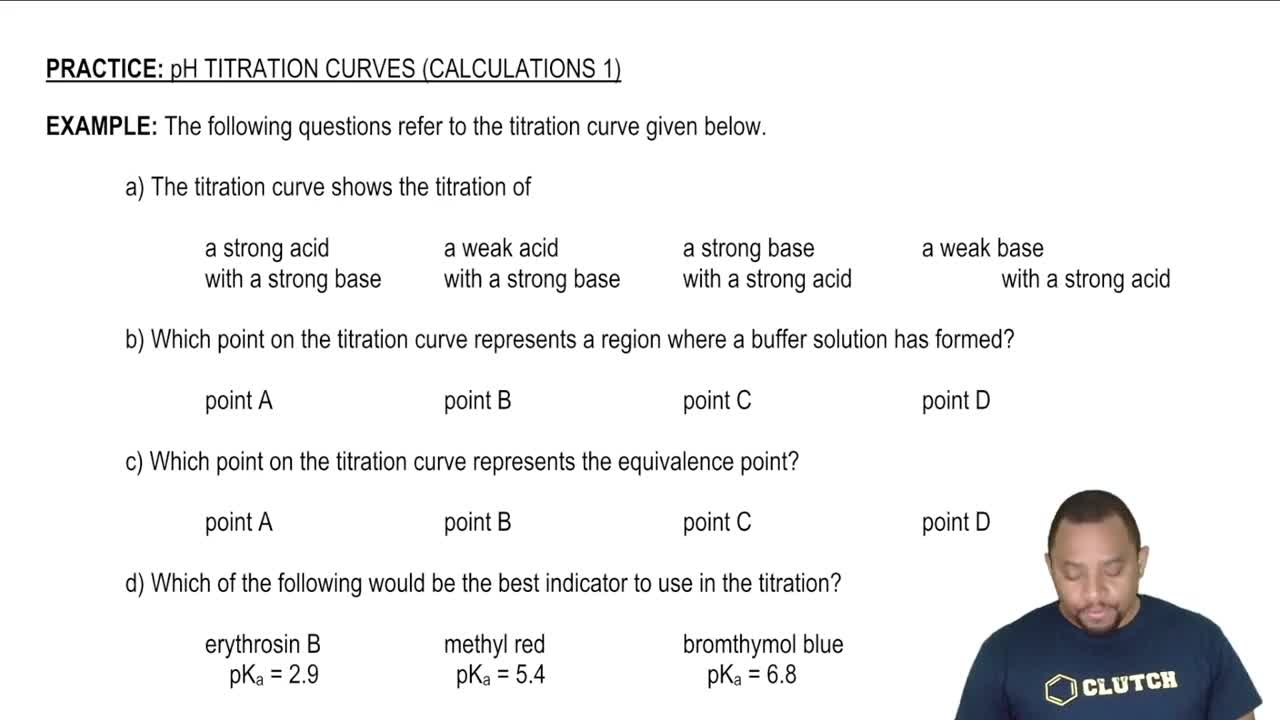06:43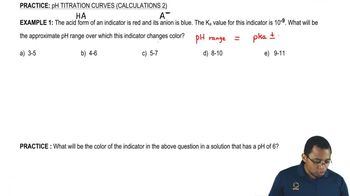03:06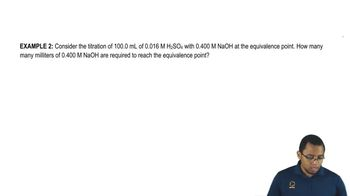06:2002:2701:27